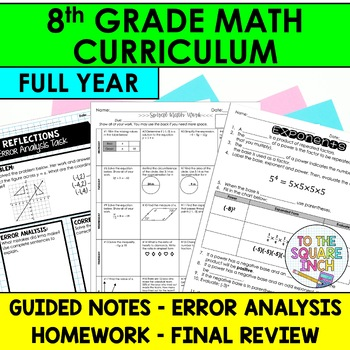DID YOU KNOW:
Seamlessly assign resources as digital activities

Learn how in 5 minutes with a tutorial resource. Try it Now

Learn More# 8th Grade Math Curriculum for Distance Learning7th - 9th
Subjects
Standards
Resource Type
Formats Included
•Google Drive™ folder
Pages
1,182 pages
\$171.82
Bundle
List Price:
\$259.75
You Save:
\$87.93
\$171.82
Bundle
List Price:
\$259.75
You Save:
\$87.93This bundle contains one or more resources with Google apps (e.g. docs, slides, etc.).

#### Products in this Bundle (76)

showing 1-5 of 76 products

#### Bonus

Digital Versions of Notes

### Description

8th Grade Math Curriculum Mega Bundle- Common Core Aligned for All Standards

This bundle includes FREE versions of digital notes for distance learning. All digital notes have been added.

This bundle includes notes, error analysis, homework and final review activities for the entire year! Included in this bundle:

This bundle includes guided notes, practice activities, warm-up and exit slips for each of the 8th Grade Math Common Core Standards. This product will include 5 units- The Number System, Equations and Expressions, Probability and Statistics, Functions and Geometry.

Expressions and Equations

• Exponents
• Multiplying Powers with the Same Base
• Power of a Power
• Power of a Product
• Dividing Exponents
• Zero and Negative Exponents
• Square Roots
• Cube Roots
• Solving Equations Using Square and Cube Roots
• Writing Scientific Notation
• Adding or Subtracting Numbers in Scientific Notation
• Multiplying Numbers in Scientific Notation
• Dividing Numbers in Scientific Notation
• Graphing Linear Equations
• Slope of Horizontal and Vertical Lines
• Slope of a Line
• Slope of Parallel and Perpendicular Lines
• Direct Variation
• X and Y Intercepts
• Graphing Linear Equations in Slope-Intercept Form
• Graphing Linear Equations in Standard Form
• Writing Equations in Slope-Intercept Form
• Writing Equations in Point-Slope Form
• Solving Simple Equations
• Combining Like Terms to Solve Equations
• Using the Distributive Property to Solve Equations
• Solving Equations with Variables on Both Sides
• Literal Equations
• Equations with No Solutions and Infinitely Many Solutions
• Solving Systems of Linear Equations by Graphing
• Solving Systems of Linear Equations by Substitution
• Solving Systems of Linear Equations by Elimination Using Addition
• Solving Systems of Linear Equations by Elimination Using Subtraction

Functions

• Relations and Functions
• Representations of Functions
• Linear Functions
• Linear and Nonlinear Functions
• Sketching Functions

The Number System

• Classifying Real Numbers
• Rational Numbers as Decimals
• Approximating Square Roots
• Comparing and Ordering Real Numbers

Geometry

• Congruent Figures
• Translations
• Reflections
• Rotations
• Composite Transformations
• Dilations
• Similar Figures
• Parallel Lines and Transversals
• Interior and Exterior Angles of Triangles
• Angles of Polygons
• Similar Triangles
• Pythagorean Theorem
• Converse of the Pythagorean Theorem
• Distance in the Coordinate Plane
• Volume of Cylinders
• Volume of Cones
• Volume of Spheres

Statistics

• Scatter Plots
• Lines of Fit
• Two-Way Frequency Tables
Total Pages
1,182 pages
Included
Teaching Duration
1 Year
Report this Resource to TpT
Reported resources will be reviewed by our team. Report this resource to let us know if this resource violates TpT’s content guidelines.

### Standards

to see state-specific standards (only available in the US).
Describe qualitatively the functional relationship between two quantities by analyzing a graph (e.g., where the function is increasing or decreasing, linear or nonlinear). Sketch a graph that exhibits the qualitative features of a function that has been described verbally.
Construct a function to model a linear relationship between two quantities. Determine the rate of change and initial value of the function from a description of a relationship or from two (𝘹, 𝘺) values, including reading these from a table or from a graph. Interpret the rate of change and initial value of a linear function in terms of the situation it models, and in terms of its graph or a table of values.
Interpret the equation 𝘺 = 𝘮𝘹 + 𝘣 as defining a linear function, whose graph is a straight line; give examples of functions that are not linear. For example, the function 𝘈 = 𝑠² giving the area of a square as a function of its side length is not linear because its graph contains the points (1,1), (2,4) and (3,9), which are not on a straight line.
Compare properties of two functions each represented in a different way (algebraically, graphically, numerically in tables, or by verbal descriptions). For example, given a linear function represented by a table of values and a linear function represented by an algebraic expression, determine which function has the greater rate of change.
Understand that a function is a rule that assigns to each input exactly one output. The graph of a function is the set of ordered pairs consisting of an input and the corresponding output.